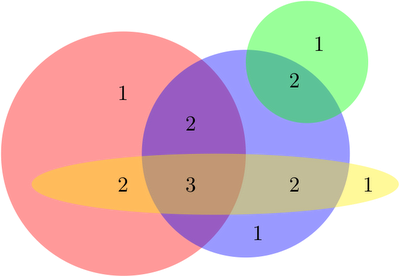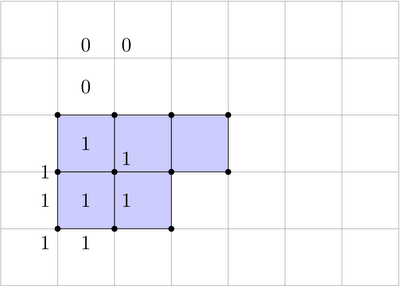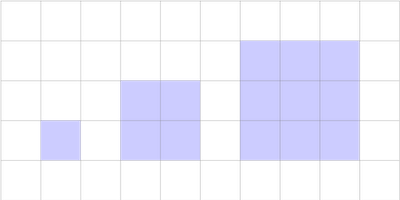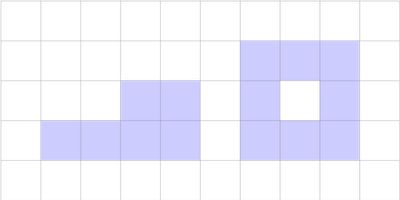## Saturday, January 16, 2010

### Target Enumeration with the Euler Characteristic. Parts 1 & 2

Part 1

`> import Data.Char`

A Statement of the Problem
The problem I ultimately want to solve, and its solution, is described in the paper Target Enumeration via Euler Characteristic Integrals. My goal here is to show how to implement that solution on a computer and make it accessible to a wider audience.

Suppose we have a set of targets we want to count. This could be anything from enemy tanks rolling over the plains to electronically tagged wildlife roaming the countryside. Each target has a region of influence which might simply be circular in shape, or might be more complex and depend on the target. Now suppose that we have a high density of sensors scattered over our domain and that each sensor can tell us how many regions of influence it lies in. Roughly speaking, each sensor counts how many targets are nearby. How do we compute how many targets we have in total?

Here's an illustration:There are four targets. The region of influence for each one is coloured making it easy to see which region is which. I've labelled each region with an integer showing how many targets can be detected in that region. The idea is that we'd have a very dense scattering of sensors in our domain, each sensor reporting an integer. In effect we'd be getting an image like a rasterised version of that picture. But we wouldn't be getting the convenient colours, just an integer per pixel.

At first it seems like a trivial problem. The sensors can all count, and if every target is in range of a sensor, every target will be counted. But we can't simply add the numbers from all of the sensors as many sensors will be in the domain of influence of the same target. If we sum all of the numbers we'll be counting each target many times over. We need to be able to subtract off the targets that are counted twice. But some targets will be counted three times and so on. And how do we tell when a target has been counted twice when all we have are counts?

We'll make one simplifying assumption in solving this problem: that the regions of influence are simply connected. In other words, they are basically some kind of shape that doesn't have holes in it. That could mean anything from a square or disk to a shape like the letter 'W'. But it excludes shapes like annuli or the letter 'B'. If we make this assumption then we can solve this problem with a very simple algorithm that will work in almost all cases. In fact, the only time it fails will be situations where no algorithm could possibly work. But there's a little ground to cover before getting to the solution.

We'll make another simplifying assumption for now. That the sensors are arranged in a rectangular grid. So the data we get back from the sensors will be a grid filled with integers. That essentially turns our problem into one of image processing and we can think of sensor values as pixels. Here's a picture where I've drawn one domain of influence and I've indicated the values returned for three of the sensors.Simple Grids
So lets assume the sensors have coordinates given by pairs of integers and that they return integer counts. The state of all the sensors can be represented by a function of this type:

`> type Field = Int -> Int -> Int`

We'll assume that we get zero if we try to read from beyond our domain. We can represent a grid of sensors, including the grid's width and height, using:

`> data Grid = Grid Int Int Field`

For efficiency something of type Field ought to read data from an array, but I'll not be assuming arrays here.

We can define display and addition of two grids:

`> instance Eq Grid> instance Show Grid where>   show (Grid w h f) = concat>       [[digit (f x y) | x <- [0..w-1]] ++ "\n" | y <- [0..h-1]]> digit 0 = '.'> digit n = chr (48+n)> instance Num Grid where>   Grid w0 h0 f0 + Grid w1 h1 f1 = Grid>       (w0 `max` w1) (h0 `max` h1)>       (\x y -> f0 x y + f1 x y)`

Ourultimate goal is to define some kind of count function with signature:

`count :: Grid -> Int`

Now suppose the function f gives the counts corresponding to one set of targets and g is the count corresponding to another. If the region of influence of these two sets of targets is separated by at least one 'pixel' then it should be clear that

`count f + count g == count (f+g)`

So at least approximately, count is additive. We also need it to be translation invariant. There's only one function that has these properties, summing up the values at all pixels:

`> gsum (Grid w h f) = sum [f x y | x <- [0..w-1], y <- [0..h-1]]`

We can implement functions to make some example grids:

`> point x y = Grid  (x+1) (y+1)>                   (\x0 y0 -> if (x0, y0) == (x,y) then 1 else 0)> circle x y r = Grid  (x+r+1) (y+r+1)>                      (\x0 y0 -> if (x-x0)^2+(y-y0)^2<r^2 then 1 else 0)`

And now we can build and display some examples:

`> test1 = circle 10 10 5+circle 7 13 4+point 5 5+point 9 12> test2 = gsum test1`

Here's a typical output:
`*Main> test1.....................................................................................1..................11111..........1111111........111111111.......111111111......1222211111.....11222221111.....11222321111.....1112222111......111122211.......1111111..........11111......................*Main> test2116`

It should be pretty clear that this doesn't count the number of targets. So how can we implement something additive and yet count targets?

Another operation we can perform on grids is scale them. Here's an implementation of scaling a grid:

`> scale n (Grid w h f) =  Grid (w*n) (h*n)>                         (\x y -> f (x `div` n) (y `div` n))`

Scaling up an `image' shouldn't change the number of targets detected. It should only correspond to the same number of targets with double-sized regions of influence. So we'd also like the following property:

`count (n `scale` f) = count f`

It's easy to see that that gsum actually has the following property for n>0:

`gsum (n `scale` f) = n^2 * gsum f`

(^ is the power function. For some reason lhs2TeX displays it as an up arrow.) These requirements are pretty tough to meet with an additive operation. But there's an amazing transformation we can perform on the data first. Instead of working on a grid with one value for each pixel we'll also store values for the 'edges' between pixels and for the 'vertices' at the corners of pixels.

Euler Grids
So lets define a new kind of grid to be a tuple of Field functions, one for faces (ie. the pixels), one for horizontal edges, one for vertical edges, and one for vertices.

`> data EGrid = EGrid {>       eWidth::Int, eHeight::Int,>       faces::Field, hedges::Field, vedges::Field, vertices::Field>   }`

The lower left vertex is (0,0) but we need to add an extra row and column of vertices on the right. Similarly we'll need an extra row and an extra column of edges. We can now `resample' our original grid onto one of these new style grids:

`> g2e (Grid w h f) = EGrid w h>   f>   (\x y  -> f (x-1)  y      `max` f x y)>   (\x y  -> f x      (y-1)  `max` f x y)>   (\x y  -> f (x-1)  (y-1)  `max` f (x-1) y `max` f x (y-1) `max` f x y)`

I'm using the rule that the value along an edge will be the maximum of the values on the two impinging faces. Similarly, the vertices acquire the maximum of the four faces they meet.

I'll try to illustrate that here:I hope you can see, from the placement of the labels, how I've attached values to edges and vertices as well as faces.

We now have a bit more freedom. We have three different types of sum we can carry out:

`> fsum (EGrid w h f _ _ _) = gsum (Grid w h f)> esum (EGrid w h _ e f _) = gsum (Grid (w+1) h e)+gsum (Grid w (h+1) f)> vsum (EGrid w h _ _ _ v) = gsum (Grid (w+1) (h+1) v)`

(We could sum over horizontal and vertical edges separately too, but if we did that then a 90 degree rotation would give a different target count.)

Now we can define a measurement function that takes three `weights' and gives us back a weighted sum:

`> measure a b c g = let e = g2e g in a*vsum e+b*esum e+c*fsum e`

We can reproduce the gsum function as

`> area = measure 0 0 1`

Try some examples to test that

`((n^2) *) . area = area . scale n`

Exercises
Now I can leave you with some challenges:

1. Find some suitable arguments a, b and c to measure so that we get:

`mystery_property1 = measure a b c((n^1) *) . mystery_property1 = mystery_property1 . scale n`

I'll let you assume that there is some choice of values that works.

(Hint: you just need to try applying mystery_property1 to a few scalings of some chosen shape. You'll quickly find some simultaneous equations in a, b and c to solve. Solve them.)

2. Can you find a simple geometric interpretation for mystery_property1? Assume that the original input grid simply consists of zeros and ones, so that it's a binary image. It shouldn't be hard to find a good interpretation. It's a little harder if it isn't a binary image so don't worry too much about that case.

3. Now find some suitable arguments a, b and c to measure so that we get:

`mystery_property2 = measure a b c((n^0) *) . mystery_property2 = mystery_property2 . scale n`

4. Can you find a simple interpretation for binary images? You might think you have it immediately so work hard to find counterexamples. Have a solid interpretation yet? And can you extend it to images that consist of more general integers?

5. Optimise the code for mystery_property2 assuming the image is binary and that the input is on a 2D array. Ultimately you should get some code that walks a 2D array doing something very simple at each pixel. Can you understand how it's managing to compute something that fits the interpretation you gave?

6. Define a version of scale called gscale that works on EGrids. Among other things we should expect:

`g2e . (scale n) == gscale n . g2e`

and that the invariance properties of the mystery properties should hold with respect to gscale.

I'll answer most of these questions in my next post. If you find mystery_property2 you've rediscovered one of the deepest notions in mathematics. Something that appears in fields as diverse as combinatorics, algebraic geometry, algebraic topology, graph theory, group theory, geometric probability, fluid dynamics, and, of course, image processing.

Part 2
The Semi-perimeter
Let's start with exercise 1 from my previous post. I allowed you to assume there was a solution. Knowing this we only need to try scaling up one shape. Here are three scalings of a single pixel:For the 1x1 square we have: 1 face, 4 edges, 4 vertices.
For the 2x2 square we have: 4 faces, 12 edges, 9 vertices.
For the 3x3 square we have: 9 faces, 24 edges, 16 vertices.

(If you don't feel like counting these yourself you can use code like:
`measure 1 0 0 \$ point 0 0measure 1 0 0 \$ 2 `scale` point 0 0measure 1 0 0 \$ 3 `scale` point 0 0`

)
and so on.

So now we have some equations:
` 4a+ 4b+ c =  x 9a+12b+4c = 2x16a+24b+9c = 3x`

We find a=0, c=-2b. I'll pick b = 1, c = -2. There's a straightfoward interpretation of measure 0 1 (-2) for binary images. It computes half of the perimeter, the semi-perimeter. There's a nice way to see this. We can try to count the number of edges in a shape starting from the number of faces in it. You can think of each face as being surrounded by 4 "half-thickness" edges. Where two faces meet we get a full thickness edge, so using half the number of faces counts internal edges correctly. But around the border of a shape we are left with contributions from only a face on one side. So we're only counting the perimeter edges by half. We get a shortfall of the semi-perimeter. Working backwards tells us how to compute the semi-perimeter from the total number of edges and faces.

For more general images, not just binary ones, we can roughly think of measure 0 1 (-2) as computing the sum of the semi-perimeters of all of the isocontours of our image.

The Euler Characteristic
The interesting case is now exercise 3. This time our equation is:
` 4a+ 4b+ c = x 9a+12b+4c = x16a+24b+9c = x`

Now we get a solution a = 1, b = -1, c = 1. If you try computing this for a few shapes it looks like it's counting the number of connected components of a binary image. However, once you realise the possibility of some holes in your image you find that it always turns out to be the total number of connected components minus the total number of holes.

Here's an example. Treat this as a single complete image:It has two components and one hole so we expect measure 1 (-1) 1 to give us 1. We can count:

14 faces
42 edges
29 vertices

Giving 29-42+14 = 1.

I'm not sure which is the easiest proof that vertices-edges+faces counts the number of components minus the number of holes. One approach is this: we only need to consider one connected component at a time. Remove its holes. Now build up the shape one pixel at a time starting with one pixel and ensuring that you have a hole-free shape at each stage. It's not hard to enumerate all of the possible ways you can add one pixel to an existing shape and show that each such addition leaves measure 1 (-1) 1 unchanged. If you now make a single pixel hole in your shape you'll see that it lowers measure 1 (-1) 1 by 1. If you now continue to add pixels to the hole, in a way that doesn't change the number of holes, you'll see that measure 1 (-1) 1 remains unchanged again.

measure 1 (-1) 1 computes what is known as the Euler characteristic of a shape. I talked a little about this in one context earlier and showed how to compute it in another context here. The Euler characteristic is a topological invariant of a shape in the sense that a rubber sheet deformation of a shape leaves the number of holes and the number of components unchanged.

The above description shows that the Euler characteristic is particularly easy to compute. It simply requires a map-reduce operation over the entire grid. But what about the separate terms: the number of components and the number of holes? These seem like simpler notions and you might expect them to be just as easy to compute. Actually they are harder to compute. Compare also with flood fill algorithms which solve a related problem. Minsky and Papert show in their book Perceptrons that any topological invariant that can be learnt by a one layer neural network (with certain resonable restrictions) must be a function of the Euler characteristic. I find it quite amazing that this notion from topology is connected (no pun intended) to learnability.

We can define

`> euler = measure 1 (-1) 1`

I have sketched an argument that euler counts #components-#holes. If we assume that each of our connected components has no holes then it counts the number of components. But here's a neat thing: if we *add* two images that contain strictly overlapping shapes (ie. not just touching each other along their boundaries) then because of additivity, euler will still count the number of shapes. In other words, if you did the exercises then you solved the target enumeration problem. It's pretty miraculous. You could splat down thousands of geometric shapes into an image. They can overlap as much as you like. But as long as they don't touch along a boundary you can still compute the total number of shapes. If two shapes do touch along a common boundary then no algorithm can work, after all they'll be indistinguishable from a single connected shape. For a quick example, notice how

`> test3 = euler test1`

recovers that test1 is the sum of 4 shapes that don't touch.

Generalised Integrals
Consider our original measurement area. This sums the values at each pixel. It is a numerical approximation to the integral of a function sampled at each pixel. Likewise each of the measure functions is numerical approximation to a generalised type of integral. The original paper uses these integrals to solve its problem. I have simply used a discrete version.

I apologise for only sketching proofs. It takes considerably more work to provide rigorous proofs. But I encourage you to experiment with the code and attempt to find counterexamples. The history of the Euler characteristic is itself characteristed by a kind of back and forth between attempted proofs and counterexamples than in a strange way mirrors the innocent looking definition: vertices-edges+faces.

By the way, some people have propsed that the Euler characteristic is a kind of generalisation of the idea of counting. It shares many properties with the usual notion of cardinality.

One last thing: I have implicitly shown that target counting is learnable by a certain type of one-layer neural network.

And thanks to @alpheccar for pointing out the original target enumeration paper.

I'm repeating this as a possibly apocryphal story I have heard from other parties: Minsky and Papert demonstrated that the only learnable topological invariants for single layer network are functions of the Euler characteristic. In particular, they demonstrated the unlearnability of connectedness. This was a precisely stated no-go theorem that discouraged and slowed investment in neural network research for many years and helped contribute to the AI Winter. Is there a good historical book on this period of AI research?Derek Elkins said...

Your statements regarding flood fill need to be a little more careful unless by "auxilary data structure" you are including a constant working memory. In the case of a color framebuffer 8 or 16 bits deep depending on whether we are considering 4- or 8-connectedness there is a linear-time, constant-space solution as described in "A Linear-Time Constant-Space Algorithm for the Boundary Fill Problem." At the cost of more time, up to Ω(n^2), but linear in the simply-connected case, you can also solve the flood fill problem in constant space as described in "Space-efficient Region Filling in Raster Graphics."

Sunday, 17 January, 2010sigfpe said...

Derek,

When I get a chance I'll tighten up what I said. In a sense flood-fill is easier because it assumes the presence of a mutable buffer which isn't being counted against the memory budget.

Sunday, 17 January, 2010sigfpe said...

Removed claim about memory usage of flood fill. I'm 100% sure there's a nice simple to state no-go theorem for counting connected components in a non-mutable image in O(1) memory, but I don't know what its precise statement is. Shouldn't have got distracted by flood-fill :-)

Sunday, 17 January, 2010D said...

On your historical addendum, the book in question is called Perceptrons (http://en.wikipedia.org/wiki/Perceptrons_(book)) I believe.

Sunday, 17 January, 2010sigfpe said...

D,

I've read Perceptrons. My interest is in the context in which that was published - and also the history of AI generally.

Sunday, 17 January, 2010Anonymous said...

Hi sigfpe!
Apologies for the off-topic post here (but I couldn't find an email address to email you at).
I'm doing a small public-domain project which involves doing some functional programming in C (mostly things like operations on arrays - head, tail, zip, fold, etc). So I was wondering - is the monad code that you did "public domain"?
( I'll certainly acknowledge that you did it anyway!).
Thanks - looking forward to your reply - bye for now :)
- Andy

Monday, 01 February, 2010sigfpe said...

If it's in my blog it's for sharing :-) But it's nice to be credited too.

Tuesday, 02 February, 2010Anonymous said...

Hi again -

Great - thanks for that!
I'll definitely credit you for the code. It's a *really neat* bit of code, too - many thanks for doing it!
Bye for now -
- Andy (mooseman)

Tuesday, 02 February, 2010Unknown said...

The colimit of your diagram is simply the Intel 4004 while the limit of the same diagram is an hypothetical infinite machine which projects onto every finite machines.

So, I guess you meant to talk about limits, instead of colimits.

Thursday, 20 May, 2010sigfpe said...

@Gery, I made a copy of your post where it's more appropriate here. You probably scrolled down too far :-)

Thursday, 20 May, 2010